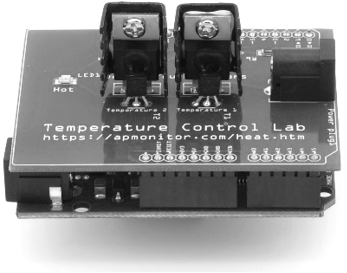## First Order Plus Dead Time (FOPDT)A first-order linear system with time delay is a common empirical description of many stable dynamic processes. The First Order Plus Dead Time (FOPDT) model is used to obtain initial controller tuning constants. An interactive FOPDT IPython Widget demonstrates the effect of the three adjustable parameters in the FOPDT equation. Downloading and running the iPython notebook locally reveals three slider bars that update the plot based on changes to the gain K_p, time constant \tau_p, and dead time \theta_p.Interactive FOPDT WidgetGitHubColab

After gaining an intuitive understanding of how the parameters influence the step response, it is important to understand the mathematical FOPDT equation. The equation

$$\tau_p \frac{dy(t)}{dt} = -y(t) + K_p u\left(t-\theta_p\right)$$

has variables y(t) and u(t) and three unknown parameters.

$$K_p \quad \mathrm{= Process \; gain}$$

$$\tau_p \quad \mathrm{= Process \; time \; constant}$$

$$\theta_p \quad \mathrm{= Process \; dead \; time}$$

#### Process Gain, K_p

The process gain is the change in the output y induced by a unit change in the input u. The process gain is calculated by evaluating the change in y(t) divided by the change in u(t) at steady state initial and final conditions.

$$K_p = \frac{\Delta y}{\Delta u} = \frac{y_{ss_2}-y_{ss_1}}{u_{ss_2}-u_{ss_1}}$$

The process gain affects the magnitude of the response, regardless of the speed of response.

#### Process Time Constant, \tau_p

Given a change in u(t) = \Delta u, the solution to the linear first-order differential (without time delay) becomes:

$$y(t) = \left( e^{-t / \tau_p} \right) y(0) + \left( 1 - e^{-t / \tau_p} \right) K_p \Delta u$$

If the initial condition y(0)=0 and at t=\tau_p, the solution is simplified to the following.

$$y\left( \tau_p \right) = \left( 1 - e^{-\tau_p / \tau_p} \right) K_p \Delta u = \left( 1 - e^{-1} \right) K_p \Delta u = 0.632 K_p \Delta u$$

Analytic Solution Derivation with Laplace Transforms

$$\tau_p \frac{dy(t)}{dt} = -y(t) + K_p u\left(t\right)$$

Perform a Laplace transform from the tables on each part of the equation:

$$\mathcal{L}\left(\tau_p \frac{dy(t)}{dt}\right) = \tau_p \left(s Y(s) - y(0)\right)$$

$$\mathcal{L}\left(-y(t)\right) = -Y(s)$$

$$\mathcal{L}\left(K_p u\left(t\right)\right) = K_p U(s)$$

If the input U(s) is a step function of size \Delta u then:

$$U(s) = \frac{\Delta u}{s}$$

Combining all of the individual Laplace transforms, the equation in Laplace domain with zero initial condition y(0)=0 is then:

$$\tau_p \, s \, Y(s) = -Y(s) + K_p \frac{\Delta u}{s}$$

At this point, the equation is algebraic and can be solved for Y(s) by combining terms on each side:

$$\tau_p \, s \, Y(s) + Y(s) = K_p \frac{\Delta u}{s}$$

and factoring out the Y(s) term:

$$Y(s) \left(\tau_p \, s + 1\right) = K_p \frac{\Delta u}{s}$$

A final steps are to isolate Y(s) on the left side of the equation and perform an inverse Laplace transform to return to the time domain:

$$Y(s) = K_p \frac{\Delta u}{s\,\left(\tau_p \, s + 1\right)}$$

$$\mathcal{L}^{-1}\left(Y(s)\right) = y(t) = K_p \left( 1-\exp \left( 1-\frac{t}{\tau_p} \right) \right) \, \Delta u$$

The process time constant is therefore the amount of time needed for the output to reach (1-exp(-1)) or 63.2% of the way to steady state conditions. The process time constant affects the speed of response.

#### Process Time Delay, \theta_p

The time delay is expressed as a time shift in the input variable u(t).

$$u\left(t-\theta_p\right)$$

Suppose that that input signal is a step function that normally changes from 0 to 1 at time=0 but this shift is delayed by 5 sec. The input function u(t) and output function y(t) are time-shifted by 5 sec. The solution to the first-order differential equation with time delay is obtained by replacing all variables t with t-\theta_p and applying the conditional result based on the time in relation to the time delay, \theta_p.

$$y(t \lt \theta_p) = y(0)$$

$$y(t \ge \theta_p) = \left( e^{-\left(t - \theta_p \right) / \tau_p}\right) y(0) + \left( 1 - e^{-\left(t - \theta_p \right) / \tau_p} \right) K_p \Delta u$$

#### Fit Lower Order Model to Data

Two popular methods to fit data or more complex models to an FOPDT systems are with a graphical method and step data or with optimization techniques.